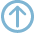# Binary encoding of GeoGig objects¶

This is the format used for internal storage in the GeoGig object store.

## Conventions¶

Formats are specified using a modified Backus-Naur notation. Definitions generally take the form:

```<structure> := part1 part2 part3
```

Indicating that the structure has three parts. The parts can be:

• Another structure, referenced by name.

• One of these specially defined structures

```NUL       := 0x00 (ASCII NUL character)
SP        := 0x20 (ASCII space character)
BR        := 0x0a (ASCII newline character)
<rev>     := <byte>* (exactly 20 bytes)
<utf8>    := <int16> <byte>* (two-byte count followed by the number
of bytes indicated by the count.
These should then be decoded as
modified UTF-8, as seen in the
readUTF and writeUTF methods in the
java.io.DataInputStream and
java.io.DataOutputStream classes
in the Java Standard Library.)
<byte>    := (8 bit byte)
<int16>   := (16 bit signed integer, "short" in Java)
<int32>   := (32 bit signed integer, "int" in Java)
<int64>   := (64 bit signed integer, "long" in Java)
<float32> := (32 bit IEEE floating point value, "float" in Java)
<float64> := (64 bit IEEE floating point value, "double" in Java)
```
• A literal byte sequence. These are generally used as markers and are represented as text in double quotes (). These markers will always be constrained to printable ASCII characters and should be encoded as ASCII, one byte per character.

• A literal byte, specified as a hexidecimal string (for example, 0xFF).

• any of the above suffixed by a modifier:

• An asterisk (*) to indicate 0 or more repetitions
• A number in brackets ([]) to indicate a specific number of repetitions.
• Comments sometimes appear to clarify the intent of certain structures. These will be enclosed in parentheses (()).

## Commit¶

```commit := commitHeader treeRef parent* authorLine committerLine message
treeRef := 0x01 <rev>
parent  := 0x02 <rev>
authorLine := 0x03 person
committerLine := 0x04 person
person := name email timestamp tzOffset
name := <utf8>
email := <utf8>
timestamp := <int64>
tzOffset := <int32>
message := <utf8>
```

## Tree¶

Note

In representing trees we split the count of tree contents into three fields: features, trees, and buckets. Because of the way GeoGig builds trees, buckets must be zero when either of the other two fields is nonzero.

We should probably document how exactly GeoGig builds trees :)

```tree := treeHeader size treeCount features trees buckets
size := <int64> (the total [recursive] count of features in this tree)
treeCount := <int32> (in a bucket tree: the number of trees that are
direct children of the bucket tree. In a node
tree: 0)
features := count node*
trees := count node*
buckets := count bucket*
count := <int32>
node := name objectId metadataId envelope nodeType
name := <utf8>
objectId := <byte>
envelope := <float64> (minx, maxx, miny, maxy.  Note that this may be
(0, -1, 0, -1) as is traditional for indicating
NULL envelopes. Of course empty (zero-area)
envelopes are valid as well.)
nodeType := <byte> (0x01: Tree, 0x02: Feature)
bucket := index objectId envelope
index := <int32>
```

## Feature¶

```feature := featureHeader count fields
count := <int32>
fields := field*
field = nullField |
booleanField | byteField | shortField | intField | longField | floatField | doubleField | stringField |
booleanArray | byteArray | shortArray | intArray | longArray | floatArray | doubleArray | stringArray |
geometryField | uuidField | bigIntField | bigDecimalField
nullField               := 0x00
booleanField            := 0x01 <byte>
byteField               := 0x02 <byte>
shortField              := 0x03 <int16>
intField                := 0x04 <int32>
longField               := 0x05 <int64>
floatField              := 0x06 <float32>
doubleField             := 0x07 <float64>
stringField             := 0x08 <utf8>
booleanArray            := 0x09 <int32> <byte>* (note that the int is the number of boolean values and booleans are packed to save space. so the number of bytes is actually the count of bits divided by 8)
byteArray               := 0x0A <int32> <byte>*
shortArray              := 0x0B <int32> <int16>*
intArray                := 0x0C <int32> <int32>*
longArray               := 0x0D <int32> <int64>*
floatArray              := 0x0E <int32> <float32>*
doubleArray             := 0x0F <int32> <float64>*
stringArray             := 0x10 <utf8>
pointField              := 0x11 <int32> <byte>* (bytes represent the geometry encoded as Well-Known Binary)
lineStringField         := 0x12 <int32> <byte>* (same)
polygonField            := 0x13 <int32> <byte>* (same)
multiPointField         := 0x14 <int32> <byte>* (same)
multiLineStringField    := 0x15 <int32> <byte>* (same)
multiPolygonField       := 0x16 <int32> <byte>* (same)
geometryCollectionField := 0x17 <int32> <byte>* (same)
geometryField           := 0x18 <int32> <byte>* (same)
uuidField               := 0x19 <int64> <int64>
bigIntField             := 0x1A <int32> <byte>*
bigDecimalField         := 0x1B <int32> <int32> <byte>* (scale, length of byte array, byte array)
datetimeField           := 0x1C <int64> (milliseconds since unix epoch)
dateField               := 0x1D <int64> (datetime with hours, minutes, seconds, milliseconds all set to 0)
timeField               := 0x1E <int64> (datetime with years, months, days all set to zero (ie, a time on Jan 1 1970))
timestampField          := 0x1F <int64> <int32> (datetime followed by a specifier of nanoseconds within the millisecond)
```

## FeatureType¶

```featureType := featureTypeHeader name properties
name := namespace localPart
namespace := <utf8>
localPart := <utf8>
properties := <int32> property*
property := name nillability minOccurs maxOccurs type
nillability := <byte> (0: non-nillable, 1: nillable. other values unused.)
minOccurs := <int32>
maxOccurs := <int32>
type := spatialType | aspatialType
aspatialType := name typeTag (aspatial types are distinguished from
spatial ones by the value of the type tag)
typeTag := <byte> (as used in features)
spatialType := name typeTag crsTextInterpretation crsText
crsTextInterpretation := <byte> (0: crsText is WKT CRS definition,
1: crsText references a well-known CRS by
identifier. If it uses URI notation
("urn:...") then the axes should be
forced to X=Easting, Y=Northing order.)
crsText := <utf8> (as determined by crsTextInterpretation)
```

## Tag¶

```tag := tagHeader objectId tagName message tagger